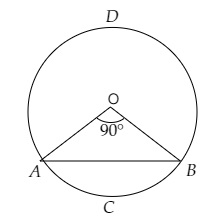# A chord of a circle of radius $14 \mathrm{~cm}$ makes a right angle at the centre. Find the areas of the minor and major segments of the circle.

Given:

A chord of a circle of radius $14 \mathrm{~cm}$ makes a right angle at the centre.

To do:

We have to find the areas of the minor and major segments of the circle.

Solution:Radius of the circle $r = 14\ cm$

Angle at the centre $\theta = 90^o$

Area of the circle $=\pi r^{2}$

$=\frac{22}{7} \times(14)^{2}$

$=616 \mathrm{~cm}^{2}$

$\mathrm{AB}$ is the chord.

Area of the minor segment $\mathrm{ACB}=(\frac{\pi \theta}{360^{\circ}}-\sin \frac{\theta}{2} \cos \frac{\theta}{2}) r^{2}$

$=(\frac{\pi \times 90^{\circ}}{360^{\circ}}-\sin \frac{90^{\circ}}{2} \cos \frac{90^{\circ}}{2})(14)^{2}$

$=(\frac{\pi}{4}-\sin 45^{\circ} \cos 45^{\circ}) \times 196$

$=196(\frac{22}{7 \times 4}-\frac{1}{\sqrt{2}} \times \frac{1}{\sqrt{2}})$

$=(\frac{22 \times 196}{28}-196 \times \frac{1}{2})$

$=154-98$

$=56 \mathrm{~cm}^{2}$

Therefore,

Area of the major segment $\mathrm{ADB}=$ Area of the circle $-$ Area of the minor segment

$=616-56$

$=560 \mathrm{~cm}^{2}$

The areas of the minor and major segments of the circle are $56\ cm^2$ and $560 \mathrm{~cm}^{2}$ respectively.

Updated on: 10-Oct-2022

86 Views